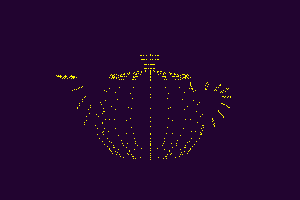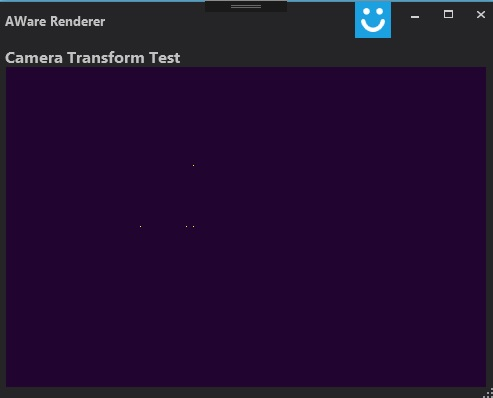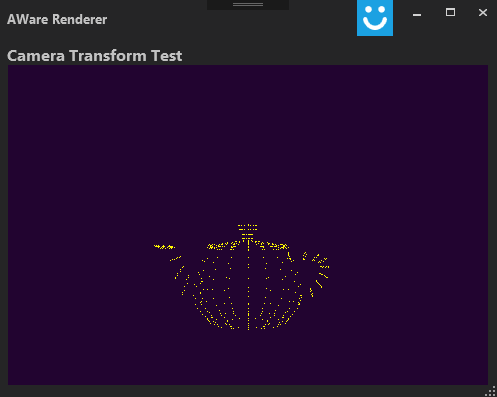# GPU Project 04 – Simple 3D object parserSo, last week we got a basic projection algorithm in place. We “rendered” the vertices of the cube into a bitmap, but we barely know got it see it working. We definitely need something more complicated to see it operating. One option is to just try to hard code a list of larger vertices that describe a more complex object, but doing that by hand is definitely cumbersome, inexact and prone to errors. Instead, I decided to rely on the vast world wide web and find several 3d objects that I could use. It turns out that there are millions of such objects in many, many websites, but all of these are in different formats. After some hunting, I setted on using a .obj format. These are the reasons:

• Plain ASCII format: Can’t beat this when it comes to ease of parsing
• No compression
• Simple 3d object structure.
• Vertexes and faces are separate.

First off, this is how a tetrahedron .obj file looks like:

```# tetrahedron.obj

g tetrahedron

v 1.00 1.00 1.00
v 2.00 1.00 1.00
v 1.00 2.00 1.00
v 1.00 1.00 2.00

f 1 3 2
f 1 4 3
f 1 2 4
f 2 3 4```

Comments use #, vertices use v and faces use f. Can’t go any simpler than that! So, I upgraded my app to use a few classes to simplify things. I now have a point class for any set of 3 numbers that should go together:

``````public class Point
{
public double x, y, z;
public Point(double x, double y, double z)
{
this.x = x;
this.y = y;
this.z = z;
}
}``````

And an object (for now) is simply a collection of points in 3d space:

``````public class Object
{
public List<Point> vertexes;
public Object()
{
vertexes = new List<Point>();
}
}``````

And I made a little snippet to open and parse the file into the structure.

``````public void loadObj(string fileName, Point origin, double scale)
{
Object obj = new Object();
string line, vertex_string;
while ((line = file.ReadLine()) != null)
{
if (line.Length > 5)
{
if (line.Substring(0, 2) == "v ") // Parse vertices
{
vertex_string = line.Substring(2);
string[] coordinates = vertex_string.Split(new char[] { ' ' }, StringSplitOptions.RemoveEmptyEntries);
double x = double.Parse(coordinates);
double y = double.Parse(coordinates);
double z = double.Parse(coordinates);
obj.vertexes.Add(new Point(x * scale - origin.x, -y * scale - origin.y, z * scale - origin.z));
}
}
}
file.Close();
}``````

This is the .obj that we just look at generates:So, if it worked with the simplest of .obj files, will it work with something else? Let’s try it out with a teapot.obj file as well:This is awesome! So the parser works, and we are able to actually “render” something on the bitmap. I think we are ready to move this into the HW.